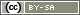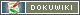[[put()]]

# put()

`void put(string variable, mixed value, [boolean EachVariable])`

The function `put` enables you to store a single value in the data record. `put()` is used when the questionnaire calculates or draws a value – and this value is needed for the analysis. For example, if the stimulus in a question is varied at random, then which one the respondent made a statement on has to be known for the analysis.

• variable
The ID of the internal variable (see below), in which the value should be saved. The ID is entered as a string in quotation marks, e.g. `'IV01_01`'.
• value
A number or text (string), which should be stored in the variable. Ordinarily, a variable with the corresponding value is given as the second parameter.
• EachVariable (optional)
Normally, `put()` only stores in internal variables. In some cases it may be useful to overwrite the value of another variable. In this case a third parameter `true` can be specified.

Warning: Only use the third parameter EachVariable if you know exactly what you are doing and an internal variable cannot be used. Changing response codes can invalidate the validity of the data set. For most cases the functions `preset()` and `dropValue()` are much better suited.

Tip: If you want to save not a single value but a whole array in the data set, use `putList()`.

## Internal Variables

Before `put()` can be used, create an internal variable in the data record. In order to do this, create a new question in the List of Questions with the type internal variables, with at least one variable (item) in it. The complete ID for this variable is entered as the first parameter in the command `put()`. The ID can be found in the Variables Overview (e.g. if it is the first variable in the first question in the “IV” category, the ID is “IV01_01”).

Tip: Items in a question with the type “internal variables” can also be used in order to save values from JavaScript. To do so, integrate the question in the same way as usual, and write the responses in the hidden input fields that are generated (Use Custom Form Elements).

## Example: Calculations

The following example assumes that one construct is assessed in two scales with the question IDs AB01 and AB02 – and that an internal variable AB03_01 was created in order to save the scale index (total value of all items).

```\$sum01 = valueSum('AB01');
\$sum02 = valueSum('AB02');
\$index = \$sum01 + \$sum02;
put('AB03_01', \$index);```

Determining the scale index during the survey is particularly useful if you want to inform the participant of the result, or if it is required for a filter:

```if (value('AB03_01') > 100) {
question('FU03');  // question for people who rate the construct higher on the scale
} elseif (value('AB03_01') > 50) { {
question('FU02');  // question for people who rate the construct averagely on the scale
} else {
question('FU01');  // question for people who rate the construct lower on the scale
}```

## Example: Transforming Codes

SoSci Survey stores positive response codes for scales (Encoding and output values). If you need the value range from -2 to +2 for a five-level scale in the data set, you can recode individual variables as follows.

Note: In practice, the recoding or transformation of values is usually part of the evaluation and is therefore performed in the statistics software. There are therefore only very special cases in which such a transformation makes sense already during the survey.

Note: To “rotate” individual items of a scale (e.g. to save a 5 instead of a 1 for “does not apply”, as with the other items), select the item on the left in the Question Catalogue and activate the option Invert answer codes for this item.

The following code transforms the answers for the scale item SK01_01 from the value range 1 to 5 into the range -2 to +2. If no valid answer was given for the scale item (item omitted or an alternative option selected), no value is stored in the data set. The PHP code is placed on the page after question SK01 (see notes on `value()`).

Precondition is that an internal variable SK02_01 has been created in advance (see above).

```\$original = value('SK01_01');
// Save only valid values (>0)
if (\$original > 0) {
put('SK02_01', \$original - 3);
} else {
put('SK02_01', false);
}```

The PHP code can also be made shorter (but less clear):

```\$org = value('SK01_01');
put('SK02_01', (\$org > 0) ? \$org - 3 : false);```

If this transformation is to be performed for all items of the scale SK01 (and sufficient internal variables have been created in SK02), the following loop does this task. The “inner” part of the PHP code is almost identical to the PHP code above. The function `id()` only creates the IDs SK01_01 to SK01_10 (for e.g. 10 items).

```\$items = getItems('SK01');
foreach (\$items as \$item) {
\$original = value(id('SK01', \$item));
if (\$original > 0) {
put(id('SK02', \$item), \$original - 3);
} else {
put(id('SK02', \$item), false);
}
}```

The following example is taken from a questionnaire that shows different questions at several points for new customers and existing customers. A new customer distinguishes himself by showing that he is yet to have bought anything (answer 1 in selection question “KD01”) and that the current order has not yet been delivered (answer 1 to 3 in selection question “KD02”).

Of course, the filter could be repeated on each page with different questions – on the one hand, however, this is unnecessarily complicated, and, on the other hand, the distinction between new and existing customers is needed in the analysis anyway. Therefore, the this should be saved in the internal variable “KD03_01”.

```if ((value('KD01') == 1) and (value('KD02') <= 3)) {
put('KD03_01', 1);  // code 1 for new customers
} else {
put('KD03_01', 2);  //code 2 for existing customers
}```

The saved code can be used on later pages for filters with no hassle.

```if (value('KD03_01') == 1) {
question('SU02', '1-3,5');  // in question SU02 show items 1, 2, 3 and 5
} else {
question('SU02', '1,2,5-7');  // in question SU02 show items 1, 2 and 5, 6, 7
}```

## Example: Randomization I

If you use `shuffle()` to create a randomly mixed list, it will not be saved at first. With `put()` you can either save the mixed list – or the ranking of the elements. Depending on what is needed for the evaluation.

The following PHP code randomly arranges the values from 1 to 10:

```\$elements = array(1,2,3,4,5,6,7,8,9,10);
shuffle(\$elements);```

The content of the mixed array `\$values` could look like this:

Index0123456789
Element72105948361

Die zugehörigen Ränge wäre dann:

Element12345678910
Rang10286491753

To save the sequence, you need a question of the type “internal variables” with the same number of variables (items) as the list has elements, e.g. for this example 10. If the question has the ID `IV01`, the values can be saved in the data set in the mixed sequence as follows:

```for (\$i=0; \$i<count(\$elements); \$i++) {
// Create internal variable ID from question ID plus index
\$id = id('IV01', \$i + 1);
// Save the value from the array here
put(\$id, \$elements[\$i]);
}```

To the index `\$i` the value one is added in the line with `id()`, because the indices of arrays start counting at 0, but the internal variables start counting at 1.

Often it is more useful to save the ranks of the elements. For example if `shuffle()` is used to mix texts, images or pages in the questionnaire randomly. You can save the ranks of the elements with the following PHP code.

```for (\$i=0; \$i<count(\$elements); \$i++) {
// Create internal variable ID from question ID plus element content (!)
\$id = id('IV01', \$elements[\$i]);
// Save the rank here
put(\$id, \$i + 1);
}```

Of course, this code only works if the elements are numerical elements that start counting with 1. To store the ranks of other array contents (e.g. page labels), you need a slightly different PHP code:

```\$pages = array('A1','A2','A3','A4','A5','B1','B2-B4','B5');
// Do not mix the pages directly, but their positions
\$indices = array_keys(\$pages);
shuffle(\$indices);
// Now save the ranks - and save the new sequence of pages in \$random
\$random = array();
for (\$i=0; \$i<count(\$indices); \$i++) {
// Save rank as above  \$id = id('IV01', \$indices[\$i] + 1);
put(\$id, \$i + 1);
// Save page in sequence
\$random[\$i] = \$pages[\$indices[\$i]];
}
// Set new page sequence
// After the last page we will continue on page "demography"
setPageOrder(\$random, 'demography');```

## Example: Randomization II

If you work with the `randomization with the `[:en:create:functions:random|random()]]' command in a randomization, you must store the random number thrown in the data set.

Important: We advise against using the function `random()` in the context of experiments. A Random Generator usually solves this task much better, see also the chapter Randomization.

The following example assumes that you have created an internal variable with the ID “IV01_01”.

```// Dice an integer between 1 and 2
\$nubmer = random(1,2);
// Save the diced number in the data set
put('IV01_01', \$number);
// And depending on it, display text module "text1" or "text2
if (\$number == 1) {
text('text1');
} else {
text('text2');
}```